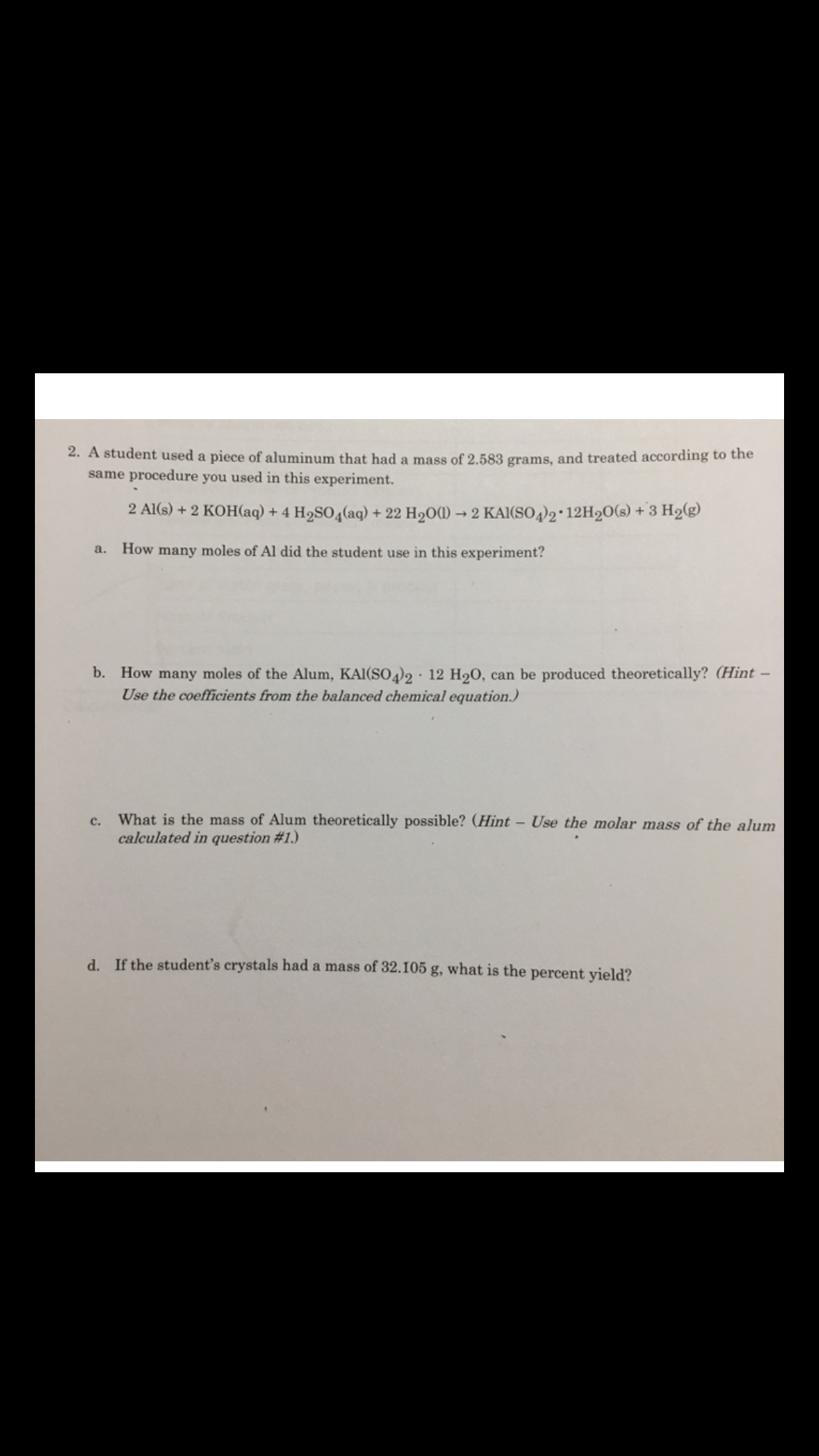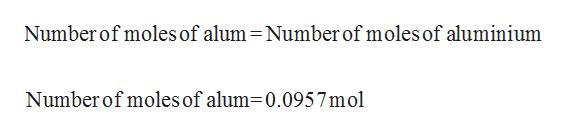# 2. A student used a piece of aluminum that had a mass of 2.583 grams, and treated according to thesame procedure you used in this experiment.2 A1(s)+2 KOH(aq) + 4 H2SO4(aq) +22 H200) 2 KAI(SO4)2 12H20(s) +3 H2(g)How many moles of Al did the student use in this experiment?a.How many moles of the Alum, KAI(SO42 * 12 H20,Use the coefficients from the balanced chemical equation.)b.can be produced theoretically? (Hint-What is the mass of Alum theoretically possible? (Hint - Use the molar mass of the alumcalculated in question #1.)c.d. If the student's crystals had a mass of 32.105 g, what is the percent yield?

Question
53 viewshelp_outlineImage Transcriptionclose2. A student used a piece of aluminum that had a mass of 2.583 grams, and treated according to the same procedure you used in this experiment. 2 A1(s)+2 KOH(aq) + 4 H2SO4(aq) +22 H200) 2 KAI(SO4)2 12H20(s) +3 H2(g) How many moles of Al did the student use in this experiment? a. How many moles of the Alum, KAI(SO42 * 12 H20, Use the coefficients from the balanced chemical equation.) b. can be produced theoretically? (Hint- What is the mass of Alum theoretically possible? (Hint - Use the molar mass of the alum calculated in question #1.) c. d. If the student's crystals had a mass of 32.105 g, what is the percent yield? fullscreen
check_circle

Step 1

The balanced equation for the given reaction is shown below:

Step 2

Given that the mass of Aluminiumis 2.583 g. Number of moles of Aluminium can be determined by the below equation:

Step 3

Ratio between the number of moles of alum and number of moles of aluminium is in the ratio 2:2 which ...help_outlineImage TranscriptioncloseNumber of moles of alum Number of moles of aluminium Number of moles of alum=0.0957mol fullscreen

### Want to see the full answer?

See Solution

#### Want to see this answer and more?

Solutions are written by subject experts who are available 24/7. Questions are typically answered within 1 hour.*

See Solution
*Response times may vary by subject and question.
Tagged in

### General Chemistry